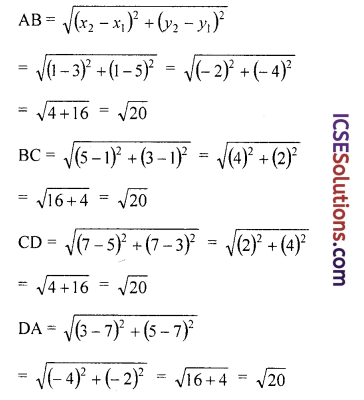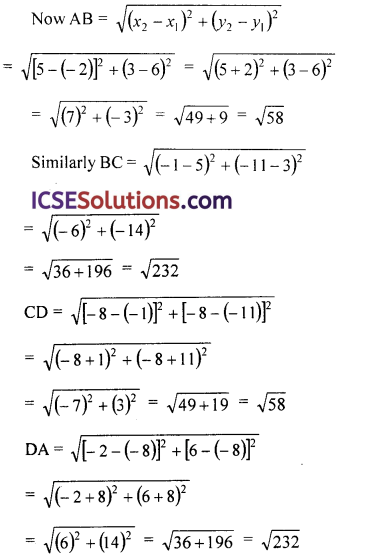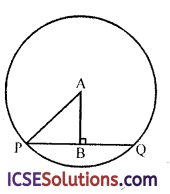The availability of ICSE S Chand Maths Class 9 Solutions Chapter 20 Coordinates and Graphs of Simultaneous Linear Equations Ex 20(D) encourages students to tackle difficult exercises.

## S Chand Class 9 ICSE Maths Solutions Chapter 20 Coordinates and Graphs of Simultaneous Linear Equations Ex 20(D)

Question 1.
Find the distance between each of the following pairs of points :
(i) (0, 0), (2, 3)
(ii) (-3, 0), (o, √7)
(iii) (a, 0), (0, a)
(iv) (7, 9), (4, 5)
(v) (-6,-1), (-6, 11)
(vi) (a + b, a – b), (a – b,-a – b)
(vii) (2, -11), (-4, -3)
(viii) (a, b), (2a, b).
Solution:
We know that distance between two points P (x1 y1) and Q (x2, y2)
= $$\sqrt{\left(x_2-x_1\right)^2+\left(y_2-y_1\right)^2}$$. Therefore

(i) Distance between (0, 0) and (2, 3)
= $$\sqrt{(2-0)^2+(3-0)^2}$$ = $$\sqrt{2^2+3^2}$$
= $$\sqrt{4+9}$$ = √13

(ii) Distance between,(-3, 0) and (0, √7)
= $$\sqrt{[0-(-3)]^2+(\sqrt{7}-0)^2}$$ = $$\sqrt{(3)^2+(\sqrt{7})^2}$$ = $$\sqrt{9+7}$$ = √16 = 4

(iii) Distance between (a, 0) and (0, a)
= $$\sqrt{(0-a)^2+(a-0)^2}$$ = $$\sqrt{a^2+a^2}$$ = $$\sqrt{2 a^2}$$ = a√2

(iv) Distance between (7, 9) and (4, 5)
= $$\sqrt{(4-7)^2+(5-9)^2}$$ = $$\sqrt{(-3)^2+(-4)^2}$$ = $$\sqrt{9+16}$$ = √25 = 5

(v) Distance between (-6, -1), (-6, 11)
= $$\sqrt{[-6-(-6)]^2+[11-(-1)]^2}$$
= $$\sqrt{(-6+6)^2+(11+1)^2}$$
= $$\sqrt{0^2+12^2}$$
= $$\sqrt{12^2}$$
= 12

(vi) Distance between (a + b, a – b) and (a – b, -a, -b)
= $$\sqrt{[(a-b)-(a+b)]^2+[(-a-b)-(a-b)]^2}$$
= $$\sqrt{(a-b-a-b)^2+(-a-b-a+b)^2}$$
= $$\sqrt{(-2 b)^2+(-2 a)^2}$$
= $$\sqrt{4 b^2+4 a^2}$$
= $$\sqrt{4\left(a^2+b^2\right)}$$
= 2$$\sqrt{a^2+b^2}$$

(vii) Distance between (2, -11) and (-4, -3)
= $$\sqrt{(-4-2)^2+[-3-(-11)]^2}$$
= $$\sqrt{(-6)^2+(-3+11)^2}$$
= $$\sqrt{(-6)^2+(8)^2}$$
= $$\sqrt{36+64}$$
= $$\sqrt{100}$$ = 10

(viii) Distance between (a, b) and (2a, b)
= $$\sqrt{(2 a-a)^2+(b-b)^2}$$
= $$\sqrt{(a)^2+(0)^2}$$
= $$\sqrt{a^2}$$ = aQuestion 2.
The distance between two points (0, 0) and (x, 3) is 5. Find x.
Solution:
We know that distance between two points
= $$\sqrt{\left(x_2-x_1\right)^2+\left(y_2-y_1\right)^2}$$
Distance between two points (0, 0) and (x, 3)
= $$\sqrt{(x-0)^2+(3-0)^2}$$
= $$\sqrt{x^2+3^2}$$
= $$\sqrt{x^2+9}$$
But distance is given = 5
∴ $$\sqrt{x^2+9}$$ = 5
Squaring both sides
x2 + 9 = 25 ⇒ x2 = 25 – 9 = 16 = (4)2
∴ x = 4

Question 3.
Find the radius of the circle whose
(i) centre is at (0, 0) and which passes through (-6, 8);
(ii) centre is at (2, 0) and which passes through (7, -12).
Solution:
(i) Radius = distance between (0, 0) and (-6, 8)
= $$\sqrt{(-6-0)^2+(8-0)^2}$$
= $$\sqrt{(-6)^2+(8)^2}$$
= $$\sqrt{36+64}$$
= $$\sqrt{100}$$
= 10 units

(ii) Radius = distance between (2, 0) and (7, -12)
= $$\sqrt{\left(x_2-x_1\right)^2+\left(y_2-y_1\right)^2}$$
= $$\sqrt{(7-2)^2+(-12-0)^2}$$
= $$\sqrt{(5)^2+(-12)^2}$$
= $$\sqrt{25+144}$$
= $$\sqrt{169}$$ = 13 units

Question 4.
Find the lengths of the sides of the triangle whose vertices are A (3, 4), B (2, -1) and C (4, -6).
Solution:
In a △ABC, vertices are
A (3, 4), B (2, -1), C (4, -6)
Length of AB = $$\sqrt{(2-3)^2+(-1-4)^2}$$
(Q distance = $$\sqrt{\left(x_2-x_1\right)^2+\left(y_2-y_1\right)^2}$$)
= $$\sqrt{(-1)^2+(-5)^2}$$ = $$\sqrt{1+25}$$ = $$\sqrt{26}$$

Length of BC = $$\sqrt{(4-2)^2+[-6-(-1)]^2}$$
= $$\sqrt{(2)^2+(-6+1)^2}$$
= $$\sqrt{(2)^2+(-5)^2}$$
= $$\sqrt{4+25}$$ = $$\sqrt{29}$$
and CA = $$\sqrt{(4-3)^2+(-6-4)^2}$$
= $$\sqrt{(1)^2+(-10)^2}$$
= $$\sqrt{1+100}$$ = $$\sqrt{101}$$Question 5.
Find the co-ordinates of the points on the x- axis which are at a distance of 10 units from the point (-4, 8).
Solution:
Let the point on x-axis be (x, 0) as it lies on
x-axis, therefore its y-coordiantes = 0
∴ d = $$\sqrt{\left(x_2-x_1\right)^2+\left(y_2-y_1\right)^2}$$
⇒ 10 = $$\sqrt{(-4-x)^2+(8-0)^2}$$
⇒ 10 = $$\sqrt{(-4-x)^2+(8)^2}$$
⇒ 10 = $$\sqrt{16+x^2+8 x+64}$$
⇒ 10 = $$\sqrt{x^2+8 x+80}$$
Squaring both sides,
100 = x2 + 8x + 80
⇒ x2 + 8x + 80 – 100 = 0
⇒ x2 + 8x – 20 = 0
⇒ x2 + 10x – 2x – 20 = 0⇒ x (x + 10) – 2 (x + 10) = 0
⇒ (x + 10) (x – 2) = 0
Either x + 10 = 0, then x = -10
or x – 2 = 0, then x = 2
∴ The points will be (-10, 0) and (2, 0)

Question 6.
What point on the y-axis is equidistant from P (0, 8) and Q (-4, 4)?
Solution:
Let point A be the required point which is equidistant from P and Q
∵ It lies on y-axis
∴ It x-coordinate = 0
Let point A be (0, y)
Using distance formula
Distance between AP and AQ are equal
∴ $$\sqrt{(0-0)^2+(y-8)^2}$$ = $$\sqrt{[0-(-4)]^2+(y-4)^2}$$
= $$\sqrt{0^2+(y-8)^2}$$ = $$\sqrt{(4)^2+(y-4)^2}$$
⇒ $$\sqrt{(y-8)^2}$$ = $$\sqrt{16+(y-4)^2}$$
Squaring boths sides,
(y – 8)2 = 16 + (y – 4)2
y2 – 16y + 64 = 16 + y2 – 8y – 16
y2 – 16y – y2 + 8y = 32 – 64
-8y = -32
⇒ y = $$\frac{-32}{-8}$$ = 4
∴ Point will be (0, 4)

Question 7.
A line is of length 10 and one end is at the point (-3, 2). If the ordinate of the other end be 10, prove that the abscissca will be 3 or -9.
Solution:
Let length of line AB = 10
One end point A (-3, 2) and let second end point B be (x, 10)
∴ AB = $$\sqrt{[x-(-3)]^2+(10-2)^2}$$
⇒ 10 = $$\sqrt{(x+3)^2+(8)^2}$$
Squaring both sides,
100 = (x + 3)2 + 64
(x + 3)2 + 64 – 100 = 0
x2 + 6x + 9 + 64 – 100 = 0
x2 + 6x – 27 = 0
⇒ x2 + 9x – 3x – 27 = 0⇒ x (x + 9) – 3 (x + 9) = 0
⇒ (x + 9) (x – 3) = 0
Either x + 9 = 0, then x = -9
or x – 3 = 0, then x = 3
∴ Abscissa = -9 or 3
Hence proved.

Question 8.
(i) Show that the points (-5, 1), (1, -1) and (1,-2) are collinear.
(ii) Find the value of p for which the points (-1, 3) (2, p) and (5, -1) are collinear.
Solution:
(i) Let A (-5, 1), B (1, -1) and C (1, -2) are the points
If sum of lengths of any two lines is equal to the third line then these points are collinear.

Now, AB = $$\sqrt{(1+5)^2+(-1-1)^2}$$
= $$\sqrt{6^2+(-2)^2}$$ = $$\sqrt{36+4}$$ = $$\sqrt{40}$$ = 2√10
BC = $$\sqrt{(1-1)^2+(-2+1)^2}$$
= $$\sqrt{0^2+(-1)^2}$$ = $$\sqrt{(-1)^2}$$ = √1 = 1

CA = $$\sqrt{(1+5)^2+(-2-1)^2}$$ = $$\sqrt{6^2+(-3)^2}$$
= $$\sqrt{36+9}$$ = $$\sqrt{45}$$ = $$\sqrt{9 \times 5}$$ = $$3 \sqrt{5}$$
We see that the points are not collinear.

(ii) ∵ Points (-1, 3), (2, p) and (5, -1) are collinear
Let A (-1, 3), B (2,p) and C (5, -1)
Now AB = $$\sqrt{(-1-2)^2+(3-p)^2}$$
= $$\sqrt{(-3)^2+(3-p)^2}$$
= $$\sqrt{9+9-6 p+p^2}$$
= $$\sqrt{p^2-6 p+18}$$
BC = $$\sqrt{(2-5)^2+(p+1)^2}$$
= $$\sqrt{(-3)^2+(p+1)^2}$$
= $$\sqrt{9+p^2+2 p+1}$$
= $$\sqrt{p^2+2 p+10}$$
and CA = $$\sqrt{(5+1)^2+(-1-3)^2}$$
= $$\sqrt{(6)^2+(-4)^2}$$ = $$\sqrt{36+16}$$ = $$\sqrt{52}$$
∵ A, B and C are collinear
∴ BC = AB + CA
$$\sqrt{p^2+2 p+10}$$ = $$\sqrt{p^2-6 p+18}$$ + $$\sqrt{52}$$
Squaring both sides
p2 + 2p + 10 = p2 – 6p + 18 + 52 + 2√52
$$\sqrt{p^2-6 p+18}$$
p2 + 2p + 10 – p2 + 6p – 18 – 52 = 2 × 2√13
$$\sqrt{p^2-6 p+18}$$
8p – 60 = 4√13$$\sqrt{p^2-6 p+18}$$
2p – 15 = $$\sqrt{13\left(p^2-6 p+18\right)}$$ (Dividing by 4)
Again squaring both sides,
(2p – 15)2 = 13 (p2 – 6p + 18)
⇒ 4p2 – 60p + 225 = 13p2 – 78p + 234
⇒ 13p2 – 78p + 234 – 4p2 + 60p – 225 = 0
⇒ 9p2 – 18p + 9 = 0
⇒ p2 – 2p + 1 = 0 (Dividing by 9)
⇒ (p – 1)2 = 0 ⇒ p – 1 = 0
∴ P = 1

Question 9.
Find the value of x such that AB = BC, where the coordinates of A, B and C are (-5, 2), (1, -2) and (x, 4) respectively.
Solution:
Points are A (-5, 2), B (1, -2) and C (x, 4)
Now AB = $$\sqrt{(-5-1)^2+(2+2)^2}$$
= $$\sqrt{(-6)^2+(4)^2}$$ = $$\sqrt{36+16}$$ = √52
BC = $$\sqrt{(1-x)^2+(-2-4)^2}$$
= $$\sqrt{(1-x)^2+(6)^2}$$ = $$\sqrt{(1-x)^2+36}$$
∵ AB = BC (given)
∴ √52 = $$\sqrt{(1-x)^2+36}$$
Squaring both sides
52 = (1 – x)2 + 36
⇒ (1 – x)2 = 52 – 36 = 16
⇒ 1 + x2 + 2x = 16
⇒ x2 + 2x + 1 – 16 = 0
⇒ x2 + 2x – 15 = 0
⇒ x2 + 5x – 3x – 15 = 0⇒ x(x + 5) – 3 (x + 5) = 0
⇒ (x + 5) (x – 3) = 0
Either x + 5 = 0, then x = -5
or x – 3 = 0, then x = 3
Hence x = 3, -5Question 10.
Prove that the triangles whose vertices are P (2, 0), Q (6, 0) and R (4, 4) is an isosceles triangles.
Solution:
We know that a triangle is an isosceles if any two sides are equal. Now P (2, 0), Q (6, 0) and R (4, 4)
Now PQ = $$\sqrt{(6-2)^2+(0-0)^2}$$ = $$\sqrt{4^2+0^2}$$ = $$\sqrt{16+0}$$ = √16 = 4
QR = $$\sqrt{(6-4)^2+(0-4)^2}$$ = $$\sqrt{(2)^2+(-4)^2}$$
= $$\sqrt{4+16}$$ = √20

RP = $$\sqrt{(4-2)^2+(4-0)^2}$$ = $$\sqrt{(2)^2+(4)^2}$$
= $$\sqrt{4+16}$$ = √20
∵ QR = RP = √20
∴ △PQR is an isosceles triangle.

Question 11.
Which of the triangles, having the following vertices, are right-angled triangles ?
(a) A (7, 0), B (6, 3) and C (12, 5)
(b) D (2, 0), E (5, 2) and F (1, 8)
(c) P (-4, 0), Q (-2, 5) and R (4, -1).
Solution:
We know that if the square of the longest side of a triangle is equal to the sum of the squares of the other two sides, then the triangle is a right angled triangle. Now
(a) A (7, 0), B (6, 3) and C (12, 5)
Length of AB = $$\sqrt{\left(x_2-x_1\right)^2+\left(y_2-y_1\right)^2}$$
= $$\sqrt{(7-6)^2+(0-3)^2}$$ = $$\sqrt{(1)^2+(-3)^2}$$
= $$\sqrt{1+9}$$ = √10
Similarly BC = $$\sqrt{(12-6)^2+(5-3)^2}$$
= $$\sqrt{(6)^2+(2)^2}$$ = $$\sqrt{36+4}$$ = √40
= $$\sqrt{4 \times 10}$$ = 2√10

and CA = $$\sqrt{(12-7)^2+(5-0)^2}$$
= $$\sqrt{(5)^2+(5)^2}$$ = $$\sqrt{25+25}$$
= √50 = $$\sqrt{25 \times 2}$$ = 5√2

We see that AB2 + BC2 = (√10)2 + (√40)2
= 10 + 40 = 50
and CA2 = (√50)2 = 50
∴ △ABC is a right angled triangle

(b) D (2, 0), E (5, 2) and F (1, 8)
Length of DE = $$\sqrt{\left(x_2-x_1\right)^2+\left(y_2-y_1\right)^2}$$
= $$\sqrt{(5-2)^2+(2-0)^2}$$ = $$\sqrt{3^2+2^2}$$
= $$\sqrt{9+4}$$ = √13
Similarly EF = $$\sqrt{(1-5)^2+(8-2)^2}$$
= $$\sqrt{(-4)^2+(6)^2}$$ = $$\sqrt{16+36}$$ = √52

FD = $$\sqrt{(2-1)^2+(0-8)^2}$$
= $$\sqrt{(1)^2+(-8)^2}$$ = $$\sqrt{1+64}$$ = √65
Here we see that,
DE2 + EF2 = (√13)2 + (√52)2 = 13 + 52 = 65
and FD2 = (√65)2 = 65
∵ DE2 + EF2 = FD2
∴ △DEF is a right angled triangle

(c) P (-4,0), Q (-2, 5) and R (4,-1)Question 12.
(i) The coordinates of the points A, B, C are (0, 4),(2, 5) and (3, 3) respectively. Prove that it is an isosceles right angled triangle. Also find its area.
(ii) Show that A (3, 5), B (1, 1), C (5, 3) and D (7, 7) are the vertices of a rhombus, is it a square ? Find its area.
Solution:
(i) Points are A (0, 4), B (2, 5), C (3, 3)
AB = $$\sqrt{\left(x_2-x_1\right)^2+\left(y_2-y_1\right)^2}$$
= $$\sqrt{(2-0)^2+(5-4)^2}$$ = $$\sqrt{(2)^2+(1)^2}$$ = $$\sqrt{4+1}$$ = √5
Similarly BC = $$\sqrt{(3-2)^2+(3-5)^2}$$
= $$\sqrt{(1)^2+(-2)^2}$$ + $$\sqrt{1+4}$$ = √5
and CA = $$\sqrt{(0-3)^2+(4-3)^2}$$
= $$\sqrt{(-3)^2+(1)^2}$$ = $$\sqrt{9+1}$$ = √10

We see that AB = BC = √5
∴ It is an isosceles triangle
and AB2 + BC2 =(√5)2 + (√5)2 = 5 + 5 = 10
and CA2 = (√10)2 =10
∵ AB2 + BC2 = CA2
∴ It is an isosceles right angle
Area of △ABC = $$\frac { 1 }{ 2 }$$ AB × BC
= $$\frac { 1 }{ 2 }$$ √5 × √5 = $$\frac { 5 }{ 2 }$$ = 2.5 square units

(ii) Points are A (3, 5), B (1, 1), C (5, 3) and D (7, 7)We see that = AB = BC = CD = DA
∴ ABCD is a rhombus or a square
Now diagonal AC = $$\sqrt{(5-3)^2+(3-5)^2}$$
= $$\sqrt{(2)^2+(-2)^2}$$ = $$\sqrt{4+4}$$ = √8
and diagonal BD = $$\sqrt{(7-1)^2+(7-1)^2}$$
= $$\sqrt{(6)^2+(6)^2}$$ = $$\sqrt{36+36}$$ = √72

∵ Diagonals are not equal
∴ It is a rhombus not a square
Now area of rhombus ABCD = $$\frac { 1 }{ 2 }$$ product of diagonals
= $$\frac { 1 }{ 2 }$$√8 × √72
= $$\frac { 1 }{ 2 }$$ $$\sqrt{576}$$
= $$\frac { 1 }{ 2 }$$ × 24 = 12 sq. units

Question 13.
Show that ABC is an equilateral triangle if A, B, C, have the following coordinates:
(i) A (1, √3), В (3, √3), C (2, 2, √3)
(ii) A (1, 1), B (-1, -1), C (-√3, √3).
Solution:
(i) Points are A (1, √3), B (3,√3), C (2, 2, √3)We see that = AB = BC = CA = 2
∴ △ABC is an equilateral triangle

(ii) A (1, 1), B (-1, -1), C (-√3, √3)We see that AB = BC = CA = √8
∴ △ABC is an equilateral triangle.Question 14.
Show that the points (-2, 6),(5, 3), (-1, -11) and (-8, -8) are the vertices of a rectangle.
Solution:
Let the vertices be A (-2, 6), B (5, 3), C (-1, -11) and D (-8, -8)We see that AB = CD and AD = BC
∴ ABCD is a rectangle.

Question 15.
The centre of a circle is at the origin and its radius is 10. Tell whether the following points lie (i) on (ii) inside or (iii) outside the circle.
(i) (6, 8)
(ii) (0, 11)
(ii) (-10, 0)
(iv) (7, 7)
(v) (-9, 4)
Solution:
Centre of the circle is origin O(0, 0) and length of the radius of the circle = 10 units
(i) Now distance between (0, 0) and (6, 8)
= $$\sqrt{\left(x_2-x_1\right)^2+\left(y_2-y_1\right)^2}$$
= $$\sqrt{(6-0)^2+(8-0)^2}$$ = 
= $$\sqrt{36+64}$$ = $$\sqrt{100}$$ = 10
∴ This point (6, 8) lies on the circle

(ii) Similarly, distance between (0, 0) and (0, 11)
= $$\sqrt{(0-0)^2+(11-0)^2}$$ = $$\sqrt{(0)^2+(11)^2}$$
= $$\sqrt{0+121}$$ = $$\sqrt{121}$$ = 11
∵ 11 > 10
∴ This point is outside the circle

(iii) Distance between (0, 0) and (-10, 0)
= $$\sqrt{(-10-0)^2+(0-0)^2}$$
= $$\sqrt{(-10)^2+(0)^2}$$ = $$\sqrt{100+0}$$ = $$\sqrt{100}$$ = 10
∴ This point lies on the circle

(iv) Distance between (0, 0) and (7, 7)
= $$\sqrt{(7-0)^2+(7-0)^2}$$ = $$\sqrt{(7)^2+(7)^2}$$
= $$\sqrt{49+49}$$ = $$\sqrt{98}$$
∵ √98 < 10
∴ This point is inside the circle

(v) Distance between (0, 0) and (-9, 4)
= $$\sqrt{(-9-0)^2+(4-0)^2}$$
= $$\sqrt{(-9)^2+(4)^2}$$
= $$\sqrt{81+16}$$ = $$\sqrt{97}$$
∵ $$\sqrt{97}$$ < 10
∴ This point is inside the circle.

Question 16.
The point P (2, -5) is mapped onto point P’ on reflection in the x-axis and Q(3, 7) is mapped onto the point Q’ on reflection in the origin. Find the length PQ and P’ Q’.
Solution:
∵ The point P (2, -5) is mapped onto point P’ in the x-axis
∴ The co-ordinates of P’ will be (2, 5)
∴ The point Q (3, 7) is mapped to Q’ in the origin
∵ Co-ordinates of Q’ will be (-3, -7)
Now length of PQ = $$\sqrt{\left(x_2-x_1\right)^2+\left(y_2-y_1\right)^2}$$
= $$\sqrt{(3-2)^2+[7-(-5)]^2}$$
= $$\sqrt{(1)^2+(7+5)^2}$$ = $$\sqrt{(1)^2+(12)^2}$$
= $$\sqrt{1+144}$$ = $$\sqrt{145}$$ units
and P’Q’ = $$\sqrt{(-3-2)^2+(-7-5)^2}$$
= $$\sqrt{(-5)^2+(-12)^2}$$
= $$\sqrt{25+144}$$ = $$\sqrt{169}$$ = 13 units

Question 17.
P and Q have coordinates (4, 1) and (2, 0). Find
(i) the image P’ of P under reflection in the y- axis.
(ii) the image Q’ of Q under reflection in the line PP’.
(iii) the length of P’Q’.
Solution:
Co-ordinates of point P and Q are (4, 1) and (2, 0) respectively
(i) P’ is the image of P (4, 1) under reflection in y-axis is
∴ Co-ordiantes of P’ will be (-4, 1)

(ii) The line joining the points P and P’ is parallel to x-axis at a distance of 1 on the positive of side y-axis
∵ Q’ is the image of Q (2, 0) in the line PP’
∴ Co-ordiantes of Q’ will be (2, 2x – y) or 2 (2 × 1 – 0) or
(2, 2 – 0) or (2, 2)

(iii) Length of P’Q’ = $$\sqrt{\left(x_2-x_1\right)^2+\left(y_2-y_1\right)^2}$$
= $$\sqrt{[2-(-4)]^2+(2-1)^2}$$
= $$\sqrt{(2+4)^2+(1)^2}$$ = $$\sqrt{(6)^2+(1)^2}$$
= $$\sqrt{36+1}$$ = $$\sqrt{37}$$ units

Question 18.
Point A(5, 1) is the centre of a circle with radius 13 units. AB is perpendicular to the chord PQ. B is (2, -3). Calculate the length of (i) AB (ii) PB (iii) PQ.Solution:
A (5, 1) is the centre of the circle
Radius of the circle OP = 13 units
AB ⊥ PQ, where PQ is a chord of the circle Co-ordinates of B are (2, -3)
∵ AB ⊥ PQ
∴ B is the mid-point of PQ

(i) Now AB = $$\sqrt{\left(x_2-x_1\right)^2+\left(y_2-y_1\right)^2}$$
= $$\sqrt{(2-5)^2+(-3-1)^2}$$
= $$\sqrt{(-3)^2+(-4)^2}$$ = $$\sqrt{9+16}$$
= $$\sqrt{25}$$ = 5 units

(ii) In right △APB,
AP2 = AB2 + PB2 ⇒ (13)2 = (5)2 + PB2
⇒ 169 = 25 + PB2 ⇒ PB2 = 169 – 25 = 144 = (12)2
∴ PB = 12 units

(iii) PQ = 2PB = 2 × 12 = 24 units